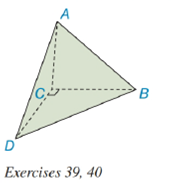Chapter 9.2, Problem 40EElementary Geometry For College St...

7th Edition
Alexander + 2 others
ISBN: 9781337614085

Solutions

Chapter
SectionElementary Geometry For College St...

7th Edition
Alexander + 2 others
ISBN: 9781337614085
Textbook Problem

Exercise 39 and 40 are based upon the “uniqueness of volume.”The volume of the pyramid shown is 32 i n 3 .a) Find the area of base △ A C D if the length of the altitude from vertex B to base △ A C D is 4 i n . b) To the nearest tenth of an inch, find the length of the altitude from vertex C to base △ A B D if A A B D ≈ 31.23 i n 2 .To determine

(a)

To find:

The area of base ACD.

Explanation

Given:

A tetrahedron (not rectangular) has vertices at A, B, C, and D. The volume of the pyramid shown is 32in3 as below,

and the length of the altitude from vertex B to base ACD is 4in.

Properties Used:

The volume V of a pyramid having a base area B and an altitude of length h is given by V=13Bh.

Area of a right-angled triangle is 12×base×height.

Approach Used:

i) Substitute the values of height h and the volume V in equation V=13Bh to find the value of area B.

Calculation:

From the given figure

To determine

(b)

To find:

The length of the altitude from vertex C to base ABD to the nearest tenth of an inch.

Still sussing out bartleby?

Check out a sample textbook solution.

See a sample solution

The Solution to Your Study Problems

Bartleby provides explanations to thousands of textbook problems written by our experts, many with advanced degrees!

Get Started

In Exercises 23-36, find the domain of the function. 25. f(x)=3x+1x2

Applied Calculus for the Managerial, Life, and Social Sciences: A Brief Approach

If P=2a+b,a=36,andb=15,findP.

Elementary Technical Mathematics

Find expressions for the quadratic functions whose graphs are shown.

Single Variable Calculus: Early Transcendentals, Volume I